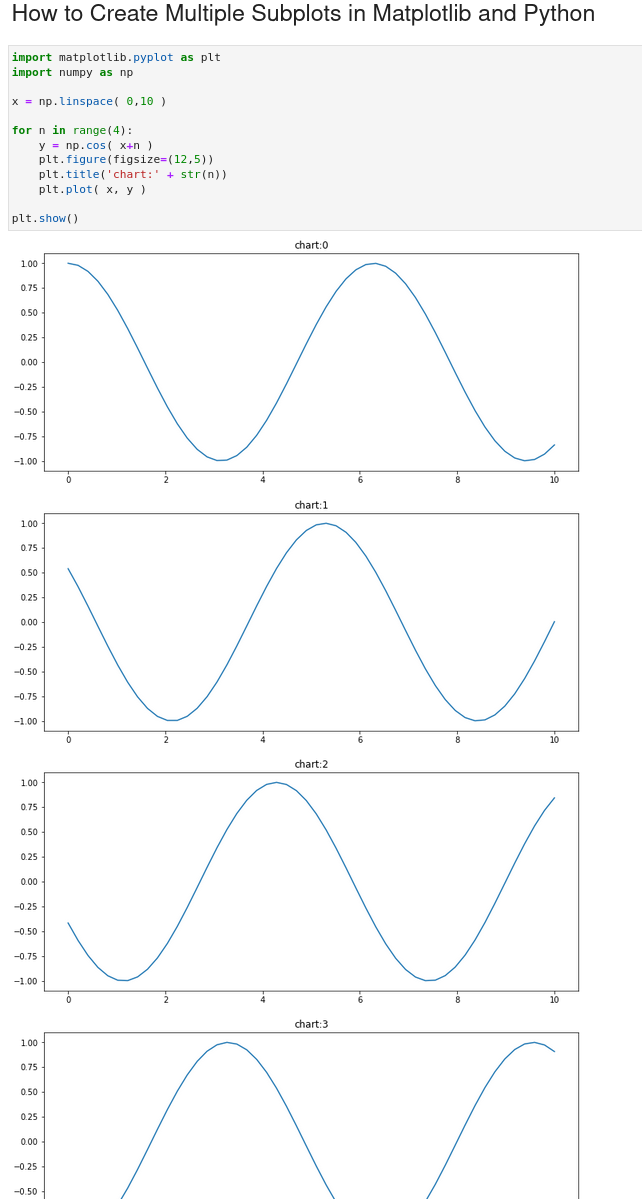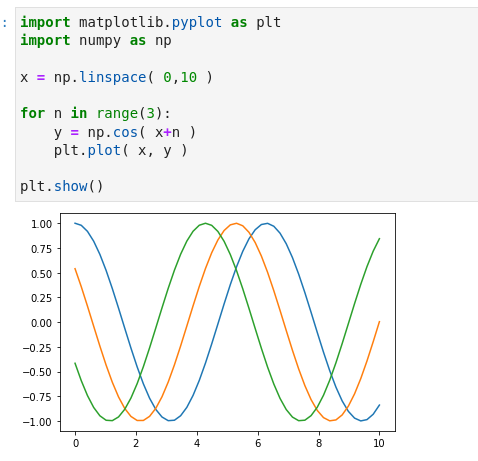To create multiple plots in Matplotlib and Python we need to use `plt.figure()` in order to create separate plots.

## Steps

• Import library - matplotlib
• Select data to be plot
• select the columns which will be used for the plot
• Loop over the selected data
• Create plot figure and select figure size
• Set title for each plot
• Select chart type
• `barplot`
• `boxplot`

## Data

For this example we will create evenly spaced numbers over interval - 0 to 10 - by `np.linspace( 0,10 )`

Then we will plot the function `np.cos()`.

The data will look like:

x y
0 0.000000 0.909297
1 0.204082 0.806088
2 0.408163 0.669421
3 0.612245 0.504970
4 0.816327 0.319561

To plot multiple plots we will increase the initial value

## Example

So to plot multiple plots with matplotlib we need to provide the `plt.figure()`

``````import matplotlib.pyplot as plt
import numpy as np

x = np.linspace( 0,10 )

for n in range(4):
y = np.cos( x+n )
plt.figure(figsize=(12,5))
plt.title('chart:' + str(n))
plt.plot( x, y )

plt.show()
``````

## Output× Note
that if
`plt.figure(figsize=(12,5))`
is missed the plots will be on a single figure